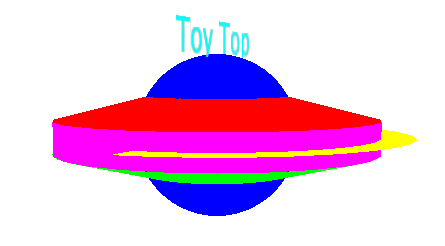Scripting Guide > Three-Dimensional Scenes > Using the Matrix Stack
Publication date: 11/29/2021

# Using the Matrix Stack

JMP 3-D scenes use a matrix stack to keep track of the current transform. The stack is initialized to the identity matrix, and each time a translate, rotate, or scale command is given, the top matrix on the stack is changed.

Note: Unlike many OpenGL implementations, JMP does not use a transposed matrix.

The JSL example below uses Push Matrix and Pop Matrix to position pieces of the toy top and then return to the origin. This is faster than using the Translate command a second time in reverse.

Figure 13.15 Drawing With a Matrix Stack`toyTop = Scene Display List();`
`toyTop << Push Matrix;`
`toyTop << Translate( 0, 0, .1 );`
`toyTop << Color( 1, 0, 0 ); // red`
` `

`toyTop << Cylinder( 1, .2, .2, 25, 5 );`
`toyTop << Pop Matrix;`
`toyTop << Push Matrix;`
`toyTop << Translate( 0, 0, -.1 );`
`toyTop << Rotate( 180, 1, 0, 0 );`
`toyTop << Color( 0, 1, 0 ); // green`
`toyTop << Cylinder( 1, .2, .2, 25, 5 );`
`toyTop << Pop Matrix;`
` `
`toyTop << Color( 0, 0, 1 ); // blue`
`toyTop << Sphere( .5, 30, 30 ); // radius, slices, stacks`
` `
`toyTop << Color( 1, 1, 0 ); // yellow`
` `

`toyTop << Partial Disk( 1, 1.2, 25, 2, 0, 270 );`
` `
`toyTop << Push Matrix;`
`toyTop << Translate( 0, 0, -.1 );`
`toyTop << Color( 1, 0, 1 ); // magenta`
`toyTop << Cylinder( 1, 1, .2, 25, 3 );`
` `

`toyTop << Pop Matrix;`
` `
`toyTop << Push Matrix;`
`toyTop << Rotate( 90, 1, 0, 0 );`
`toyTop << Translate( 0, .5, 0 );`
`toyTop << Color( 0, 1, 1 ); // cyan`
`toyTop << Text( "center", "baseline", .2, "Toy Top" );`
`toyTop << Pop Matrix;`
` `
`scene = Scene Box( 600, 600 ); // make a scene box...holds an OpenGL scene`
` `
`New Window( "Example 3", scene ); // put the scene in a window`
`scene << Perspective( 45, 3, 7 );`
`scene << Translate( 0.0, 0.0, -4.5 );`
`scene << Rotate( -85, 1, 0, 0 );`
`scene << Rotate( 65, 0, 0, 1 );`
`scene << Call List( toyTop );`
` `
`scene << Update; // update the display box`

There are some cases where you want to replace the current matrix on the stack. For these cases, use the Load Matrix command.

`Load Matrix(m)`

where m is a 4x4 JMP matrix that is loaded onto the current matrix stack.

Similar is the Mult Matrix command

`Mult Matrix(m)`

When the Mult Matrix command is issued, the matrix on the top of the current matrix stack is multiplied by m.

The following matrices perform some simple commands.

Translation:

` [1  0  0  x`
` 0  1  0  y`
` 0  0  1  z`
` 0  0  0  1]`

In the following rotation matrices, c = cos(angle) and s=sin(angle).

` [1  0  0  0`
` 0  c -s  0`
` 0 -s  c  0`
` 0  0  0  1]`

` [c  0  s  0`
` 0  1  0  0`
`-s  0  c  0`
` 0  0  0  1]`

` [c -s  0  0`
` s  c  0  0`
` 0  0  1  0`
` 0  0  0  1]`

For example, here are two equivalent (except for the translation being opposite) ways to translate and rotate a display list.

// first way uses matrix

`gl << Push Matrix;`
`xt = Identity( 4 ); // translate this one left by .75`
`xt[1, 4] = -.75;`
`xr = Identity( 4 ); // rotate this one, cos needs radians, not degrees`
`xr[2, 2] = Cos( 3.14159 * a / 180 );`
`xr[2, 3] = -Sin( 3.14159 * a / 180 );`
`xr[3, 2] = Sin( 3.14159 * a / 180 );`
`xr[3, 3] = Cos( 3.14159 * a / 180 );`
`yr = Identity( 4 );`
`yr[1, 1] = Cos( 3.14159 * a / 180 );`
`yr[1, 3] = Sin( 3.14159 * a / 180 );`
`yr[3, 1] = -Sin( 3.14159 * a / 180 );`
`yr[3, 3] = Cos( 3.14159 * a / 180 );`
`zr = Identity( 4 );`
`zr[1, 1] = Cos( 3.14159 * a / 180 );`
`zr[1, 2] = -Sin( 3.14159 * a / 180 );`
`zr[2, 1] = Sin( 3.14159 * a / 180 );`
`zr[2, 2] = Cos( 3.14159 * a / 180 );`
` `

// order of multiplication matters with matrices

`gl << Mult Matrix( xt * xr * yr * zr );`
`gl << Arcball( dl, 1 );`
`gl << Pop Matrix;`
` `

// second way uses functions

`gl << Push Matrix;`
`gl << Translate( .75, 0, 0 ); // translate this one right by .75`
`gl << Rotate( a, 1, 0, 0 ); // rotate this one in degrees`
`gl << Rotate( a, 0, 1, 0 ); // order of operations also matters here`
`gl << Rotate( a, 0, 0, 1 );`
`gl << Arcball( dl, 1 );`
`gl << Pop Matrix;`

It is not possible to read back the current transform matrix, because the matrix exists only while the display list is drawing, not while your JSL script is creating it. If you must know its content, create it in JSL and use Load Matrix to put it on the stack.